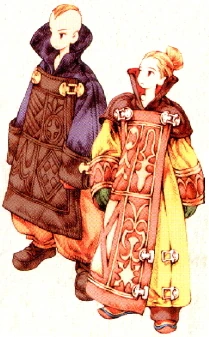# Math "Magic"? Field of Study

Good morning GWJers

I wanted to reach out to the hive mind to see if there is any field regarding odd relationships with or properties of numbers. I was hoping for something more scientific than numerology or perhaps numerology is more scientific than I fear?

What spurred this is some random thoughts about partial fractions of 11ths (i.e. not 11/11 or 0/11).
I know it is weird the things you think about as you are falling asleep! So I became riveted that 11ths are infinitely repeating and their digits add up to 9:

1/11 = .09090909 etc.
2/11 = .18181818
3/11 = .27272727
4/11 = .36363636
5/11 = .45454545
6/11 = .54545454
7/11 = .63636363
8/11 = .72727272
9/11 = .81818181
10/11 = .90909090

It also brought me back to my childhood arithmetic where we learned tricks like if something is divisible by 3 or 9 , then its digits add up to a number divisible by 3 or 9 respectively:

541311 is divisible by 3 because 5+4+1+3+1+1 = 15, which is divisible by 3
276534 is divisible by 9 because 2+7+6+5+3+4 = 27 which is divisible by 9

Is there a field of study surrounding this? I know I am not the only GWJer obsessed with, for lack of a better description - "stupid number tricks" ;P

The field of study would be "Number Theory." A quick Google search brought up this PDF which may interest you.

The youtube channel Numberphile has lots of examples of this, check it out:

Thank you, thank you!

I think those are less about number theory and more about properties that emerge in a base-10 decimal representation.

As an example, 1/10 is 0.1 in decimal, but in binary, it's an infinitely repeating number: 0.00011001100110011....

Link to the math if you want to see it.

Number theory is still probably of interest to you though.

See? I would have thought it was "Mathemagics"

Shows how much I know.

The idea that infinity exists between 1 and 2 freaks me out a little.

The outfit you get for entering the field is pretty sweet, though: